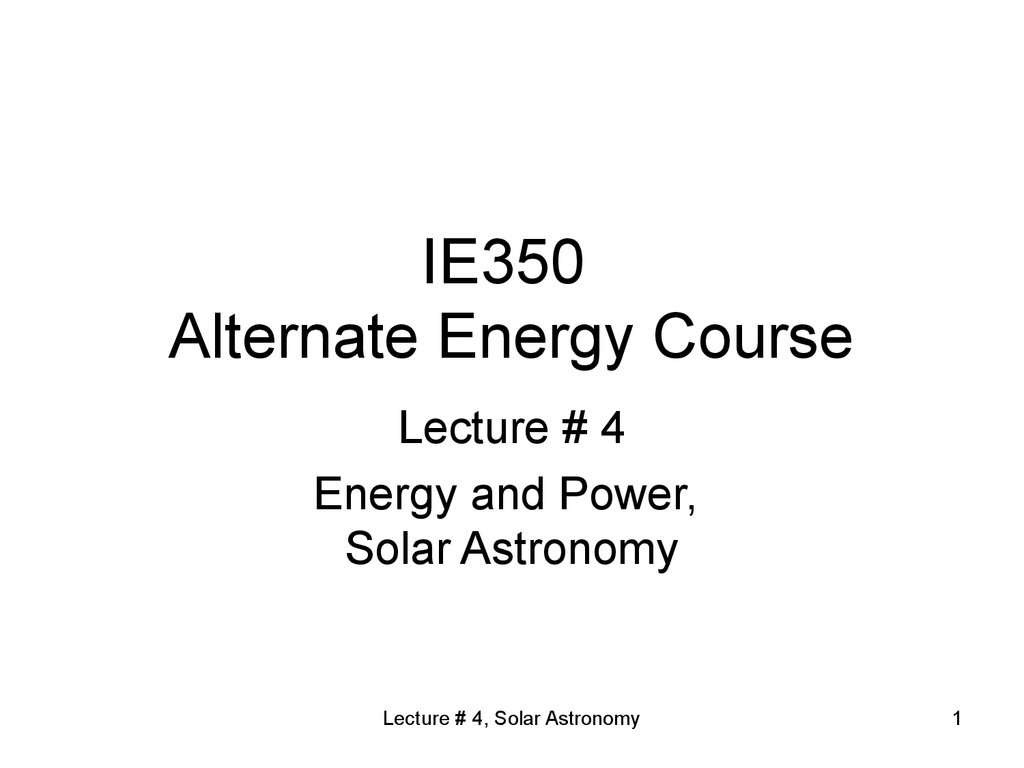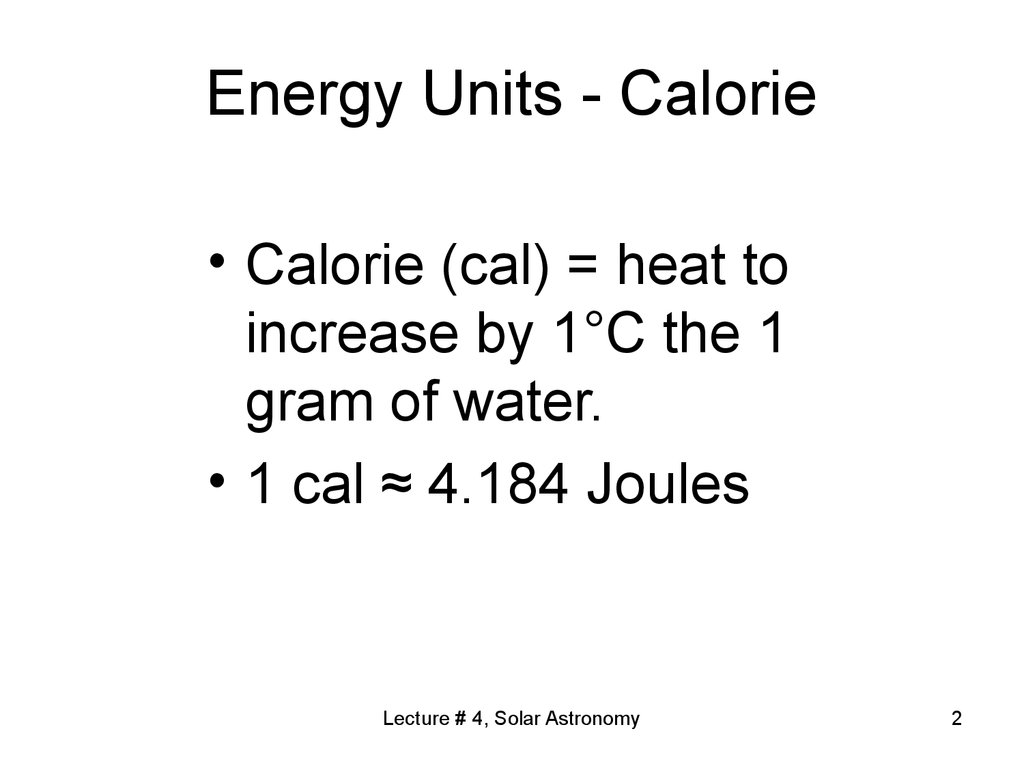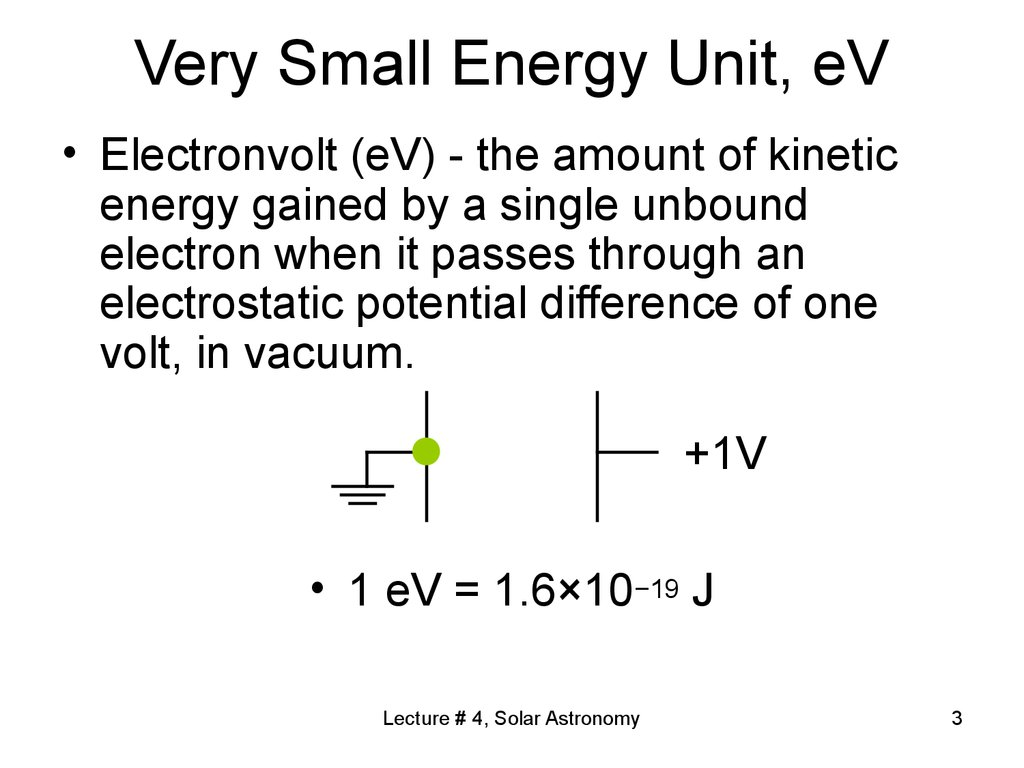# Energy and power, solar astronomy. (Lecture 4)

## 1. IE350 Alternate Energy Course

Lecture # 4
Energy and Power,
Solar Astronomy
Lecture # 4, Solar Astronomy
1

## 2. Energy Units - Calorie

• Calorie (cal) = heat to
increase by 1°C the 1
gram of water.
• 1 cal ≈ 4.184 Joules
Lecture # 4, Solar Astronomy
2

## 3. Very Small Energy Unit, eV

• Electronvolt (eV) - the amount of kinetic
energy gained by a single unbound
electron when it passes through an
electrostatic potential difference of one
volt, in vacuum.
+1V
• 1 eV = 1.6×10−19 J
Lecture # 4, Solar Astronomy
3

## 4. Energy unit conversion factors

Lecture # 4, Solar Astronomy
4

## 5. Energy and Power

• If power is constant
E = P · t, P = E/t
• If power is variable and
depends on time
E = ∫P(t)dt,
P(t) = dE(t)/dt
Lecture # 4, Solar Astronomy
5

## 6. Power Units

• Watt (W) = using one J in
one second.
• kW = 1000 W
• Horsepower = 735 W
= 0.735 kW
• MW = 1000 kW
Lecture # 4, Solar Astronomy
6

## 7. Power vs. Energy

• Thus, power is the rate of the energy use.
• Energy is what you pay for repeatedly, as
much as you use the energy, the kWh-s –
variable, operational cost.
• Power is the capacity to use the energy
• You pay for the capacity usually upfront,
fixed or installation cost.
• E.g. if you decide to buy an air conditioner,
you need to solve a power sizing problem.
You pay the fixed amount. Later you usually
use only a fraction of the total capacity.
Lecture # 4, Solar Astronomy
7

## 8. Solar Energy

• The SUN:
• Fusion in the sun – the process
• Temperature of the suncrust, black-body
• Photon energy, light speed, duality
• Electromagnetic Spectrum
• Solar constant = 1366 W/m2.
Lecture # 4, Solar Astronomy
8

## 9. The light: particle, wave

• Particle and wave
• Light speed, c = 299,792,458 m/s
c ≈ 300,000 km/s
• Photon energy, E = h = frequency,
• h is Planck’s constant, h = 6.626 10 -34 J s
h = 4.135 10-15 eV s.
• = c/
• E = hc/
Lecture # 4, Solar Astronomy
9

## 10. Electromagnetic Spectrum

Lecture # 4, Solar Astronomy
10

## 11. Sun Spectrum

Lecture # 4, Solar Astronomy
11

## 12. The Sun

• Sun has a capacity of 3.86×1026 W
3.86×108 EJ/s
• Earth gets only two-billionth part of it.
• 127,400,000 km² - Earth cross-section
• 1.740 1017 W = 0.174 EJ/s
• Armenian annual energy consumption:
• Solar Constant
=1366 W/sq.m.
• Average Insolation
= ¼ of solar const.
= 342 W/sq.m.
Lecture # 4, Solar Astronomy
12

## 13. How this energy is generated?

Lecture # 4, Solar Astronomy
13

## 14. How this energy is generated?

• About 74% of the Sun's
mass is hydrogen, 25% is
helium, and the rest is
quantities of heavier
elements.
Lecture # 4, Solar Astronomy
14

## 15. How this energy is generated?

• The Sun has a surface
temperature of
approximately 5,500 K,
giving it a white color, which,
because of atmospheric
scattering, appears yellow.
Lecture # 4, Solar Astronomy
15

## 16. How this energy is generated?

• The Sun diameter:
1.4 106 km = 109 that of the
earth.
• Distance from Earth:
1.5 108 km, = 8.31 min at
light speed
Lecture # 4, Solar Astronomy
16

## 17. How this energy is generated?

It was Albert Einstein who
provided the essential
clue to the source of the
Sun's energy output with
his mass-energy relation:
E=mc²
Lecture # 4, Solar Astronomy
17

## 18.

Lecture # 4, Solar Astronomy
18

## 19.

Lecture # 4, Solar Astronomy
19

## 20. The Sun

Lecture # 4, Solar Astronomy
20

## 21.

Lecture # 4, Solar Astronomy
21

## 22.

Lecture # 4, Solar Astronomy
22

## 23.

Lecture # 4, Solar Astronomy
23

## 24. NASA caption: Giant magnetic loops dance on the sun’s horizon in concert with the eruption of a solar flare—seen as a bright flash of light—in this imagery from NASA’s Solar Dynamics Observatory, captured Jan. 12-13, 2015. Image Credit: NASA/SDO

NASA caption: Giant magnetic loops dance on
the sun’s horizon in concert with the eruption
of a solar flare—seen as a bright flash of light
—in this imagery from NASA’s Solar Dynamics
Observatory, captured Jan. 12-13, 2015.
Image Credit: NASA/SDO
Lecture # 4, Solar Astronomy
24

## 25.

Lecture # 4, Solar Astronomy
25

## 26.

Lecture # 4, Solar Astronomy
26

## 27.

Lecture # 4, Solar Astronomy
27

## 28. Sun surface videos

v=ipvfwPqh3V4
v=0WW1HN0iG0M
v=lpzCSZ7Eerc
v=nmDZhQAIeXM
Lecture # 4, Solar Astronomy
28

## 29. Solar wind

• The total number of particles carried away
from the Sun by the solar wind is about
1.3×1036 per second.
• Thus, the total mass loss is about
4–6 billion tons per hour.
• Composed of:
- electrons,
- protons
- alpha particles
Lecture # 4, Solar Astronomy
29

## 30. Elementary particles flow from Sun – Solar Wind http://www.independent.co.uk/travel/europe/watch-this-beautiful-timelapse-of-the-northern-lights-over-norway-9735690.html http://www.bbc.com/news/science-environment-28690559 https://www.youtube.com/watch?

Elementary particles flow from Sun
– Solar Wind
Aurora Borealis
Lecture # 4, Solar Astronomy
30

## 31. Solar Wind

Lecture # 4, Solar Astronomy
31

## 32. Aurora Borealis

v=hsMW7zbzsUs
v=Vdb9IndsSXk
v=pjgvGiEHlNs
Lecture # 4, Solar Astronomy
32

## 33. How this energy is generated?

In 1920 Sir Arthur Eddington
proposed that the pressures and
temperatures at the core of the
Sun could produce a nuclear
fusion reaction that merged
hydrogen into helium, resulting in
a production of energy from the
net change in mass.
Lecture # 4, Solar Astronomy
33

## 34.

This actually corresponds
to a surprisingly low
rate of energy
production in the Sun's
µW/cm³ (microwatts
6 µW/kg of matter.
For comparison, the
human body produces
heat at approximately
the rate 1.2 W/kg,
roughly a million times
greater per unit mass.
Lecture # 4, Solar Astronomy
34

## 35.

Lecture # 4, Solar Astronomy
35

## 36. How this energy is generated?

most of the elements
been created by
nuclear reactions
inside stars like the
Sun.
Lecture # 4, Solar Astronomy
36

## 37. 1.5 The future of energy resources

• Solar Constant = 1366 W/sq.m.
• Sahara’s surface area = 9,000,000 sq.km.
• If we use 10% of Sahara with 12.5%
efficiency, we will get 1000 Exajoules/year!
• This is twice as much as current world
consumption.
• I can see the future «Ocean Solar Power
Plants», that produce Hydrogen!
• However, population grows exponentially!
Lecture # 4, Solar Astronomy
37

## 38.

Lecture # 4, Solar Astronomy
38

## 39. Earth's rotation

• Earth's rotation tilts about 23.5 degrees on
its pole-to-pole axis, relative to the plane
of Earth's solar system orbit around our
sun.
• As the Earth orbits the sun, this creates
the 47-degree peak solar altitude angle
difference, and the hemisphere-specific
difference between summer and winter.
Lecture # 4, Solar Astronomy
39

## 40.

Lecture # 4, Solar Astronomy
40

## 41. Solar Constant

Planets, Distances and Incidences
Planet
(AU)
Incidence
(W/m2)
Mercury
Venus
Earth/Moon
Mars
0.387
0.720
1.000
1.520
9,121
2,635
1,366
591
Asteroid Belt
2.500
219
3.5 AU Distance
3.500
112
Jupiter
5.190
51
7 AU Distance
7.000
28
Saturn
Uranus
Neptune
Pluto
9.510
19.000
30.000
39.480
15
4
1.5
0.9
Lecture # 4, Solar Astronomy
41

## 42. Now: go to the article http://www.wired.com/2015/07/pluto-new-horizons-2/

Lecture # 4, Solar Astronomy
42

## 43.

Lecture # 4, Solar Astronomy
43

## 44. Solar radiation bouncing atmosphere

the theoretical daily-average insolation
atmosphere
at the top of the atmosphere, where θ
is the polar angle of the Earth's orbit,
and θ = 0 at the vernal equinox, and
θ = 90° at the summer solstice; φ is
the latitude of the Earth. The
calculation assumed conditions
appropriate for 2000 A.D.: a solar
constant of S0 = 1367 W m−2,
obliquity of ε = 23.4398°, longitude of
perihelion of ϖ = 282.895°,
eccentricity e = 0.016704. Contour
labels (green) are in units of W m−2
Lecture # 4, Solar Astronomy
44

## 45.

Lecture # 4, Solar Astronomy
45

## 46. Airmass

• In astronomy, airmass is the optical path
length through Earth's atmosphere for light
from a celestial source.
• As it passes through the atmosphere, light
is attenuated by scattering and absorption;
the more atmosphere through which it
passes, the greater the attenuation.
• Consequently, celestial bodies at the
horizon appear less bright than when at the
zenith.
Lecture # 4, Solar Astronomy
46

## 47. Earth Atmosphere

Lecture # 4, Solar Astronomy
47

## 48. Rayleigh scattering

Lecture # 4, Solar Astronomy
48

## 49. Airmass

• “Airmass” normally indicates relative
airmass, the path length relative to that at
the zenith at sea level, so by definition, the
sea-level airmass when the sun is at the
zenith is 1.
• Airmass increases as the angle between the
source and the zenith increases, reaching a
value of approximately 38 at the horizon.
• Airmass can be less than one at an elevation
greater than sea level.
Lecture # 4, Solar Astronomy
49

## 50. Airmass

Atmosphere height = 8.5 ÷ 11 km.
Earth's mean radius is 6371 km.
Airmass abbreviation: AM##.
E.g. at angle of approximately 60 degrees over
horizon we have AM2, = 62% of solar constant.
The solar panels are often rated at AM1.5
The maximum airmass at horizon is:
AM35.5 ÷ AM39
At sea level, AM1 attenuates @ 27%.
At AM10 we have 23X attenuation
At AM20 we have >10000X attenuation
Lecture # 4, Solar Astronomy
50

## 51. Earth Atmosphere

Lecture # 4, Solar Astronomy
51

## 52. Numbers to remember

• Solar constant = 1366W/m2
• Attenuation at AM1 = 27%
• Scattered light capacity
= 1366W/m2 x 27% = 369W/m2
• Intensity at AM1 = 1366W/m2 - 369W/m2
= 997W/m2 ≈ 1000W/m2
• Reference Intensity = 1000W/m2
Lecture # 4, Solar Astronomy
52

## 53. Air mass calculations

Lecture # 4, Solar Astronomy
53

## 54. Notion of the Cost per peak watt installed

• “Peak Watt” = 1000W = 1kW
• Is the power produced at normal incidence
of solar radiation @ 1000W/m 2.
• \$/Wp - Easy way to compare various solar
conversion devices.
• Mostly useful for electric power generation
devices, such as for: Hydro; PV; Wind,
Solar Thermal Electric, etc.
Lecture # 4, Solar Astronomy
54